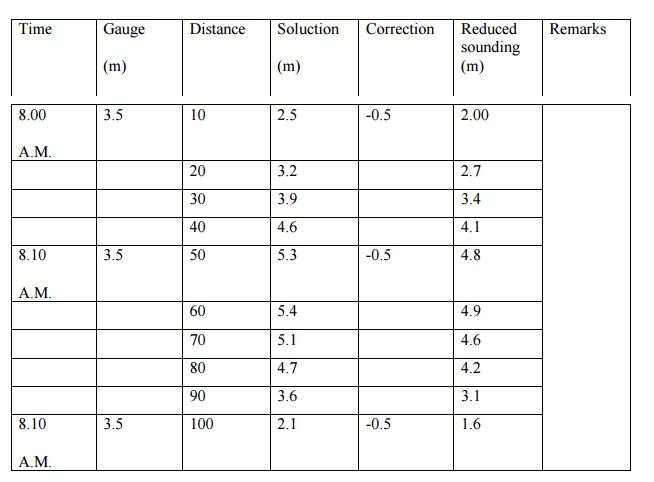Home | | Surveying | Reduction of soundings with a example

# Reduction of soundings with a example

The reduced soundings are the reduced levels of the sub-marine surface in terms of the adopted datum. When the soundings are taken, the depth of water is measured with reference to the existing water level at that time.

Reduction of soundings with a example

The reduced soundings are the reduced levels of the sub-marine surface in terms of the adopted datum. When the soundings are taken, the depth of water is measured with reference to the existing water level at that time. If the gauge readings are also taken at the same time, the soundings can be reduced to a common unvarying datum. The datum most commonly adopted is the 'mean level of low water of spring tides' and is written either as

L.W.O.S.T. (low water, ordinary spring tides) or

M.L.W.S. (mean low water springs). For reducing the soundings, a correction equal to the difference of level between the actual water level (read by gauges) and the datum is applied to the observed soundings, as illustrated in the table given below :

Gauge Reading at L.W.O.S.T. = 3.0 m.What is three point problem ?How it can be solved ?

Given the three shore signals A, B and C, and the angles ? and ? subtended by AP, BP

and CP at the boat P, it is required to plot the position of P

1. Mechanical Solution

(i) By Tracing Paper

Protract angles ? and ? between three radiating lines from any point on a piece of tracing paper. Plot the positions of signals A, B, C on the plan. Applying the tracing paper to the plan, move it about until all the three rays simultaneously pass through A, B and C. The apex of the angles is then the position of P which can be pricked through.

(ii) By Station Pointer :

The station pointer is a three-armed protractor and consists of a graduated circle with fixed arm and two movable arms to the either side of the fixed arm. All the three arms have beveled or fiducial edges. The fiducial edge of the central fixed arm corresponds to the zero of the circle. The fiducial edges of the two moving arms can be set to any desired reading and can be clamped in position. They are also provided with verniers and slow motion screws to set the angle very precisely. To plot position of P, the movable arms are clamped to read the angles ? and ? very precisely. The station pointer is then moved on the plan till the three fiducial edges simultaneously touch A, B and C. The centre of the pointer then represents the position of P which can be recorded by a prick mark.

2. Graphical Solutions

(a) First Method :

Let a, b and c be the plotted positions of the shore signals A, B and C respectively and let ? and ? be the angles subtended at the boat. The point p of the boat position p can be obtained as under :

1.     Join a and c.

2.     At a, draw ad making an angle ? with ac. At c, draw cd making an angle ? with ca. Let both these lines meet at d.

3.     Draw a circle passing through the points a, d and c.

4.     Join d and b, and prolong it to meet the circle at the point p which is the required position of the boat.

Proof. From the properties of a circle,

apd = acd = ?                       and    cpd = cad = ?

which is the required condition for the solution.

(b) Second Method :

Join ab and bc.

1.     From a and b, draw lines ao1 and bo1 each making an angle (90 o - ?) with ab on the side towards p. Let them intersect at 01.

2.     Similarly, from b and c, draw lines ================ each making an angle (90 o - ?) with ab on the side towards p. Let them intersect at --.

With - as the centre, draw a circle to pass through a and b. Similarly, with - as the centre draw a circle to pass through b and c. Let both the circles intersect each other at a point p. p is then the required position of the boat.

Proof.         ao1b  = 180 o - 2 (90 o - ?) = 2?

apb    = �    ao1b = ?

Similarly,    bo2c  = 180 o - 2 (90 o - ?) = 2?

And   bpc    = �    bo2c = ?.

The above method is sometimes known as the method of two intersecting circles.

(c)Third Method :

1.    Join ab and bc.

2.    At a and c, erect perpendiculars ad and ce.

3.    At b, draw a line bd subtending angle (90 o - ?) with ba, to meet the perpendicular through a in d.

4.    Similarly, draw a line be subtending an angle (90 o - ?) with bc, to meet the perpendicular through c in e.

5.    Join d and e.

6.    Drop a perpendicular on de from b. The foot of the perpendicular (i.e. p) is then the required position of the boat.

Study Material, Lecturing Notes, Assignment, Reference, Wiki description explanation, brief detail
Civil Surveying : Advanced Topics in Surveying : Reduction of soundings with a example |